Introduction to Reduction Notation

Reduction notation can be used to represent differences in sums of squares (SS) for two models. The notation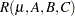denotes the complete main-effects model for effects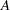,
, and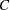. The notation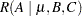denotes the difference between the model SS for the complete main-effects model containing,, andand the model SS for the reduced model containing onlyand.

In other words, this notation represents the differences in model SS produced by

```proc glm;
class a b c;
model y = a b c;
run;
```

and

```proc glm;
class b c;
model y = b c;
run;
```

As another example, consider a regression equation with four independent variables. The notation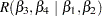denotes the differences in model SS between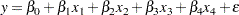and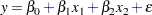This is the difference in the model SS for the models produced, respectively, by

```model y = x1 x2 x3 x4;
```

and

```model y = x1 x2;
```

The following examples demonstrate the ability to manipulate the symbolic representation of a generating set. Note that any operations performed on the symbolic notation have corresponding row operations that are performed on the generating set itself.